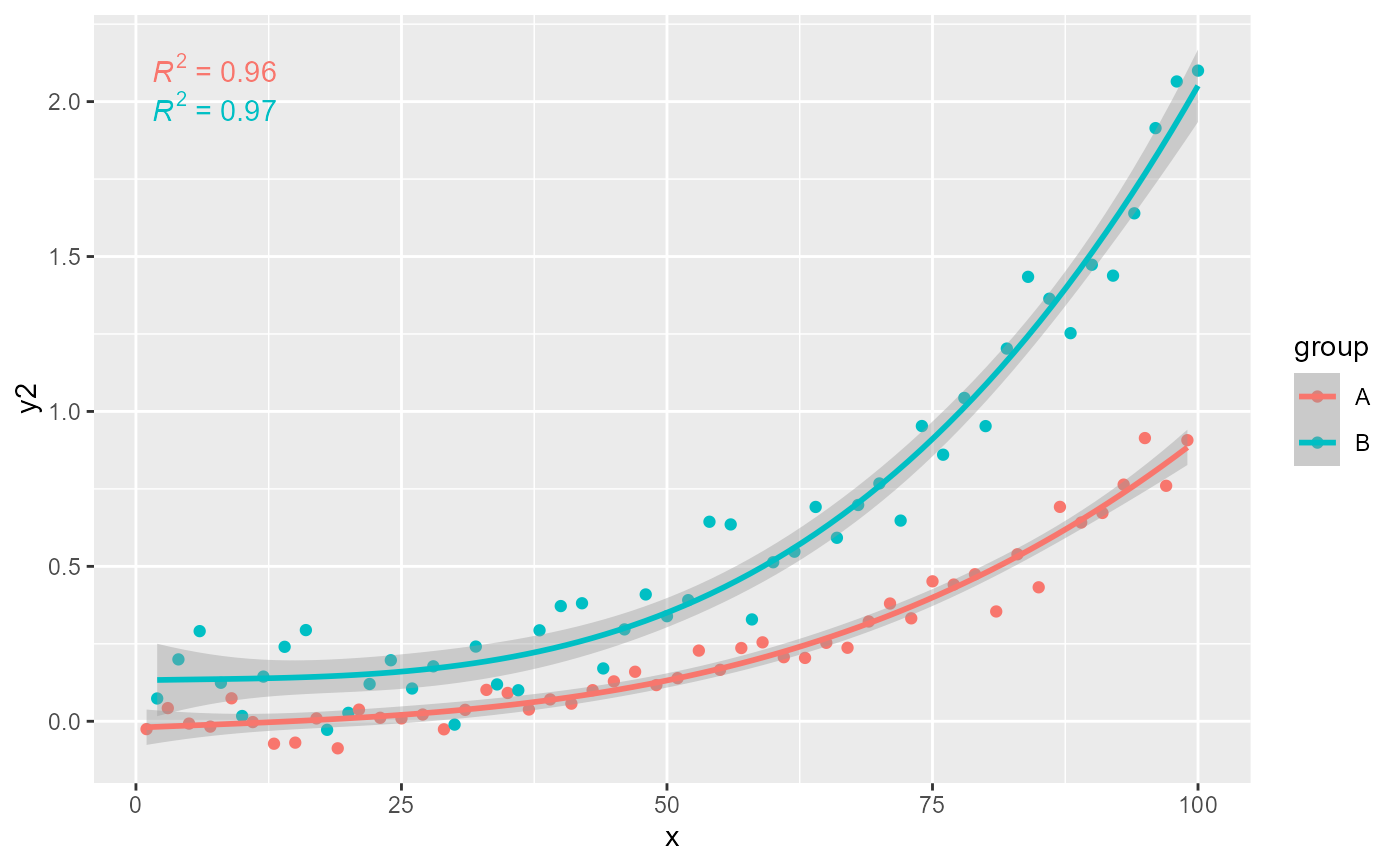stat_poly_eq fits a polynomial and generates several labels including the equation and/or p-value, coefficient of determination (R^2), 'AIC' or 'BIC'.

stat_poly_eq(mapping = NULL, data = NULL, geom = "text_npc",
position = "identity", ..., formula = NULL,
eq.with.lhs = "italic(y)~=~", eq.x.rhs = NULL, coef.digits = 3,
rr.digits = 2, label.x = "left", label.y = "top",
label.x.npc = NULL, label.y.npc = NULL, hstep = 0, vstep = NULL,
output.type = "expression", na.rm = FALSE, show.legend = FALSE,
inherit.aes = TRUE)

Arguments

mapping The aesthetic mapping, usually constructed with aes or aes_. Only needs to be set at the layer level if you are overriding the plot defaults. A layer specific dataset - only needed if you want to override the plot defaults. The geometric object to use display the data The position adjustment to use for overlapping points on this layer other arguments passed on to layer. This can include aesthetics whose values you want to set, not map. See layer for more details. a formula object. If character the string is pasted to the front of the equation label before parsing or a logical (see note). character this string will be used as replacement for "x" in the model equation when generating the label before parsing it. integer Number of significant digits to use in for the vector of fitted coefficients and for $R^2$ labels. numeric with range 0..1 "normalized parent coordinates" (npc units) or character if using geom_text_npc() or geom_label_npc(). If using geom_text() or geom_label() numeric in native data units. If too short they will be recycled. numeric with range 0..1 (npc units) DEPRECATED, use label.x and label.y instead; together with a geom using npcx and npcy aesthetics. numeric in npc units, the horizontal and vertical step used between labels for different groups. character One of "expression", "LaTeX" or "text". a logical indicating whether NA values should be stripped before the computation proceeds. logical. Should this layer be included in the legends? NA, the default, includes if any aesthetics are mapped. FALSE never includes, and TRUE always includes. If FALSE, overrides the default aesthetics, rather than combining with them. This is most useful for helper functions that define both data and aesthetics and shouldn't inherit behaviour from the default plot specification, e.g. borders.

Details

This stat can be used to automatically annotate a plot with R^2, adjusted R^2 or the fitted model equation. It supports only linear models fitted with function lm(). The R^2 and adjusted R^2 annotations can be used with any linear model formula. The fitted equation label is correctly generated for polynomials or quasi-polynomials through the origin. Model formulas can use poly() or be defined algebraically with terms of powers of increasing magnitude with no missing intermediate terms, except possibly for the intercept indicated by "- 1" or "-1" in the formula. The validity of the formula is not checked in the current implementation, and for this reason the default aesthetics sets R^2 as label for the annotation. This stat only generates the label, the predicted values need to be separately added to the plot, so to make sure that the same model formula is used in all steps it is best to save the formula as an object and supply this object as argument to the different statistics.

Note

For backward compatibility a logical is accepted as argument for eq.with.lhs, giving the same output than the current default character value. By default "x" is retained as independent variable as this is the name of the aesthetic. However, it can be substituted by providing a suitable replacement character string through eq.x.rhs.

Aesthetics

stat_poly_eq understands x and y, to be referenced in the formula and weight passed as argument to parameter weights of lm(). All three must be mappeed to numeric variables. In addition the aesthetics undertood by the geom used ("text" by default) are understood and grouping respected.

Computed variables

x

x position for left edge

y

y position near upper edge

eq.label

equation for the fitted polynomial as a character string to be parsed

rr.label

$$R^2$$ of the fitted model as a character string to be parsed

Adjusted $$R^2$$ of the fitted model as a character string to be parsed

AIC.label

AIC for the fitted model.

BIC.label

BIC for the fitted model.

hjust

Set to zero to override the default of the "text" geom.

Warning!

if using output.type = "expression", then parse = TRUE is needed, while if using output.type = "LaTeX" parse = FALSE, the default of geom_text and geom_label, should be used.

Examples

library(ggplot2)
# generate artificial data
set.seed(4321)
x <- 1:100
y <- (x + x^2 + x^3) + rnorm(length(x), mean = 0, sd = mean(x^3) / 4)
my.data <- data.frame(x = x, y = y,
group = c("A", "B"),
y2 = y * c(0.5,2),
w = sqrt(x))
# give a name to a formula
formula <- y ~ poly(x, 3, raw = TRUE)
# no weights
ggplot(my.data, aes(x, y)) +
geom_point() +
geom_smooth(method = "lm", formula = formula) +
stat_poly_eq(formula = formula, parse = TRUE)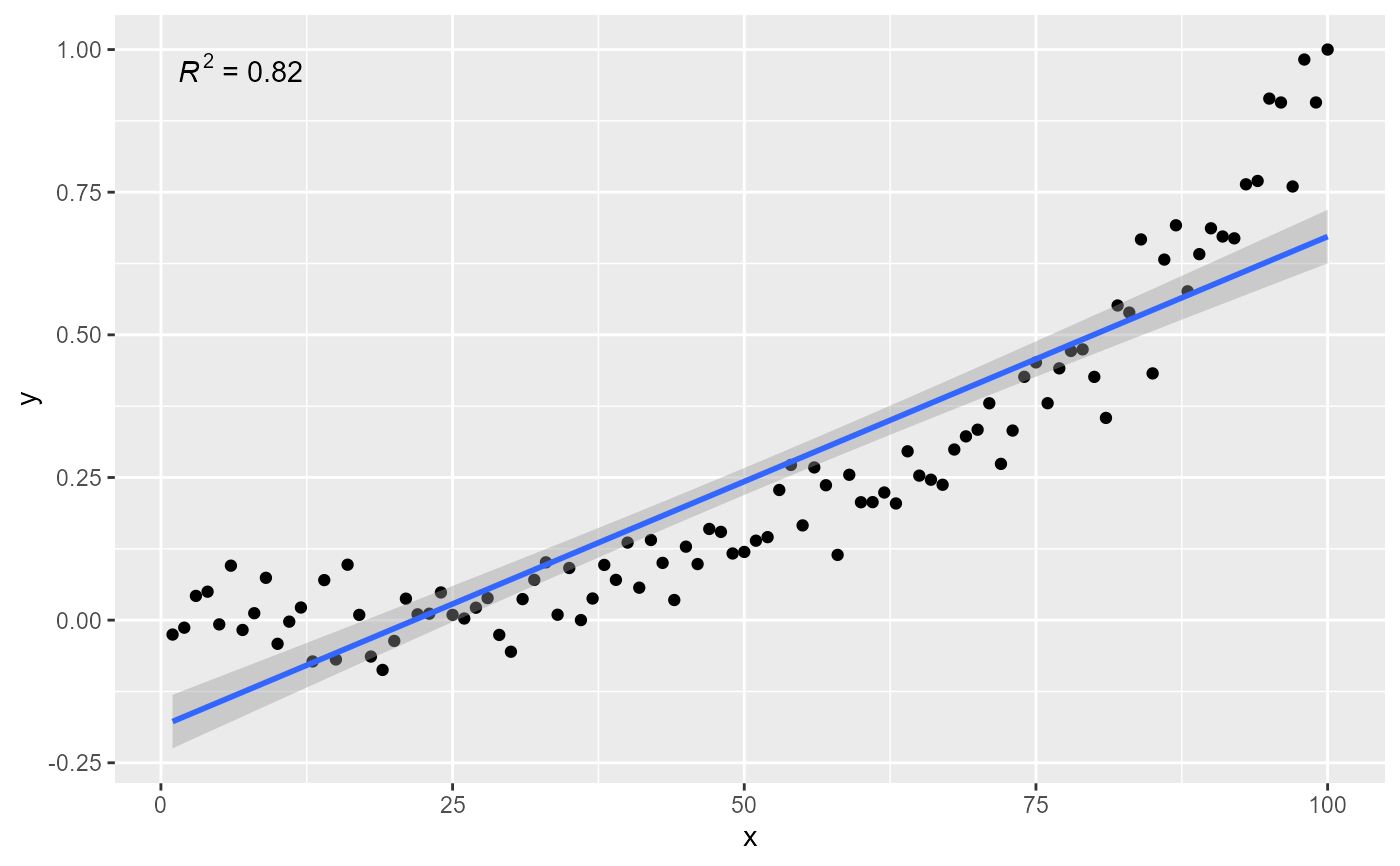ggplot(my.data, aes(x, y)) +
geom_point() +
geom_smooth(method = "lm", formula = formula) +
stat_poly_eq(formula = formula, parse = TRUE,
label.y = "bottom", label.x = "right")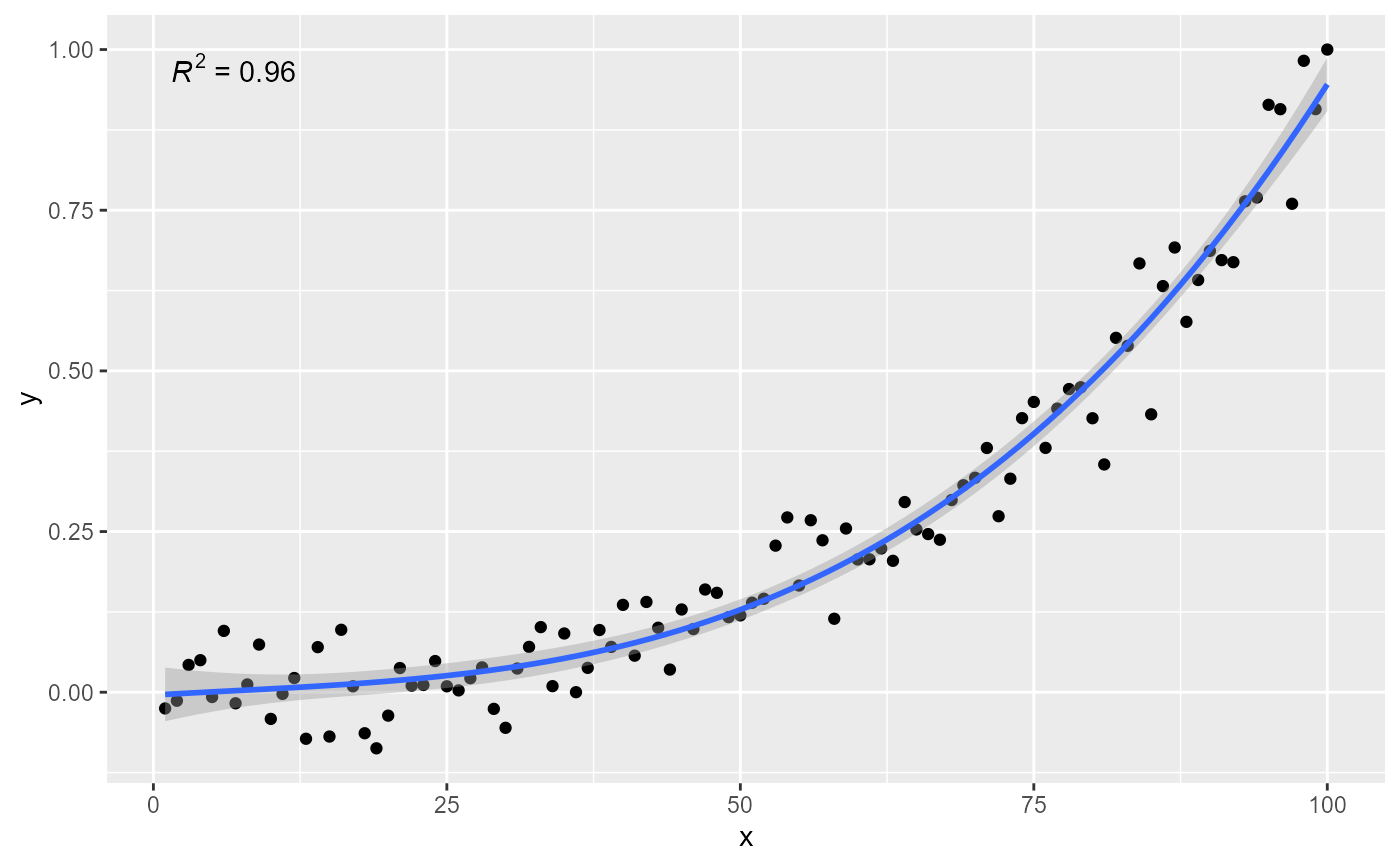ggplot(my.data, aes(x, y)) +
geom_point() +
geom_smooth(method = "lm", formula = formula) +
stat_poly_eq(formula = formula, parse = TRUE,
label.y = 0.1, label.x = 0.9)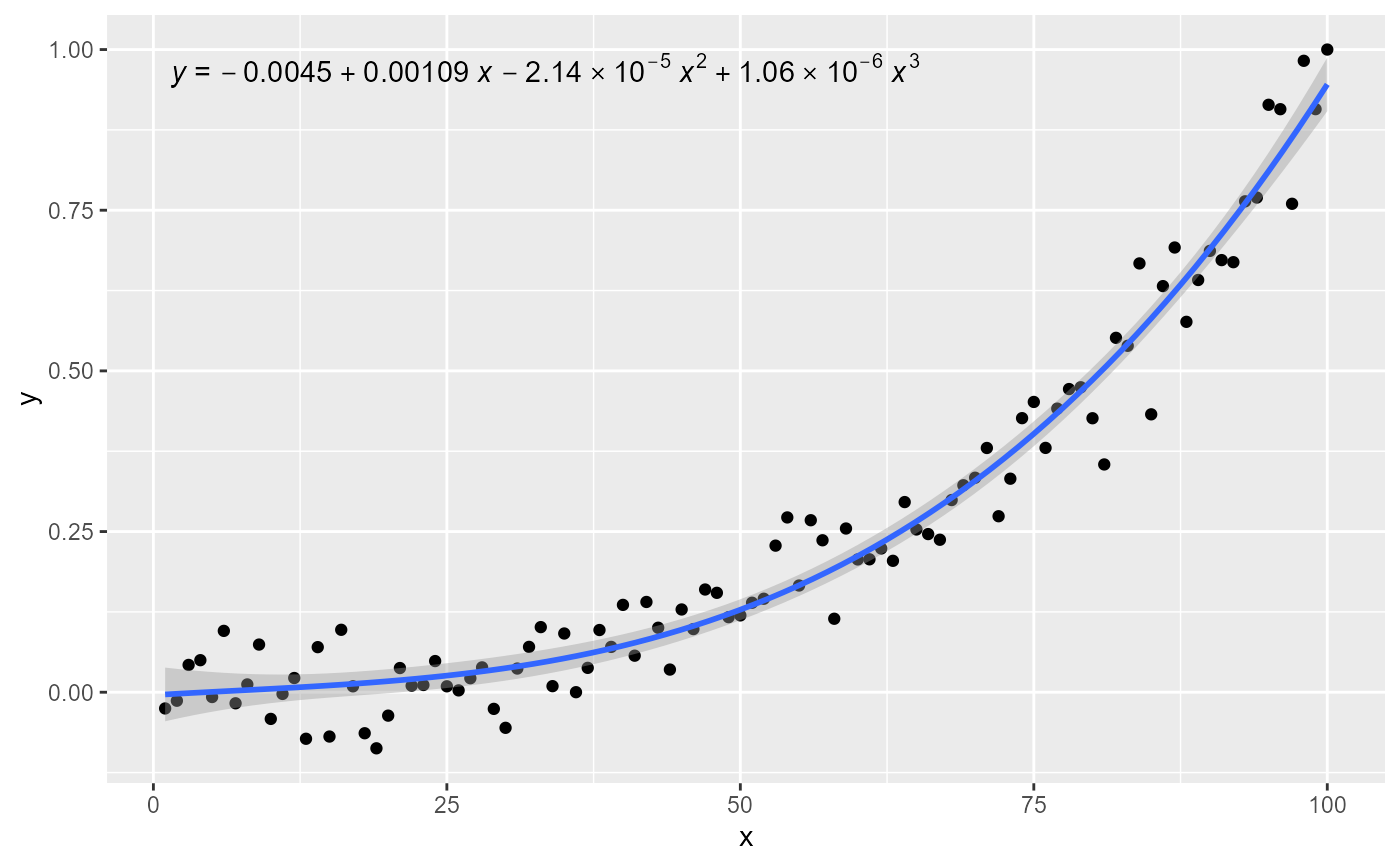# using weights
ggplot(my.data, aes(x, y, weight = w)) +
geom_point() +
geom_smooth(method = "lm", formula = formula) +
stat_poly_eq(formula = formula, parse = TRUE)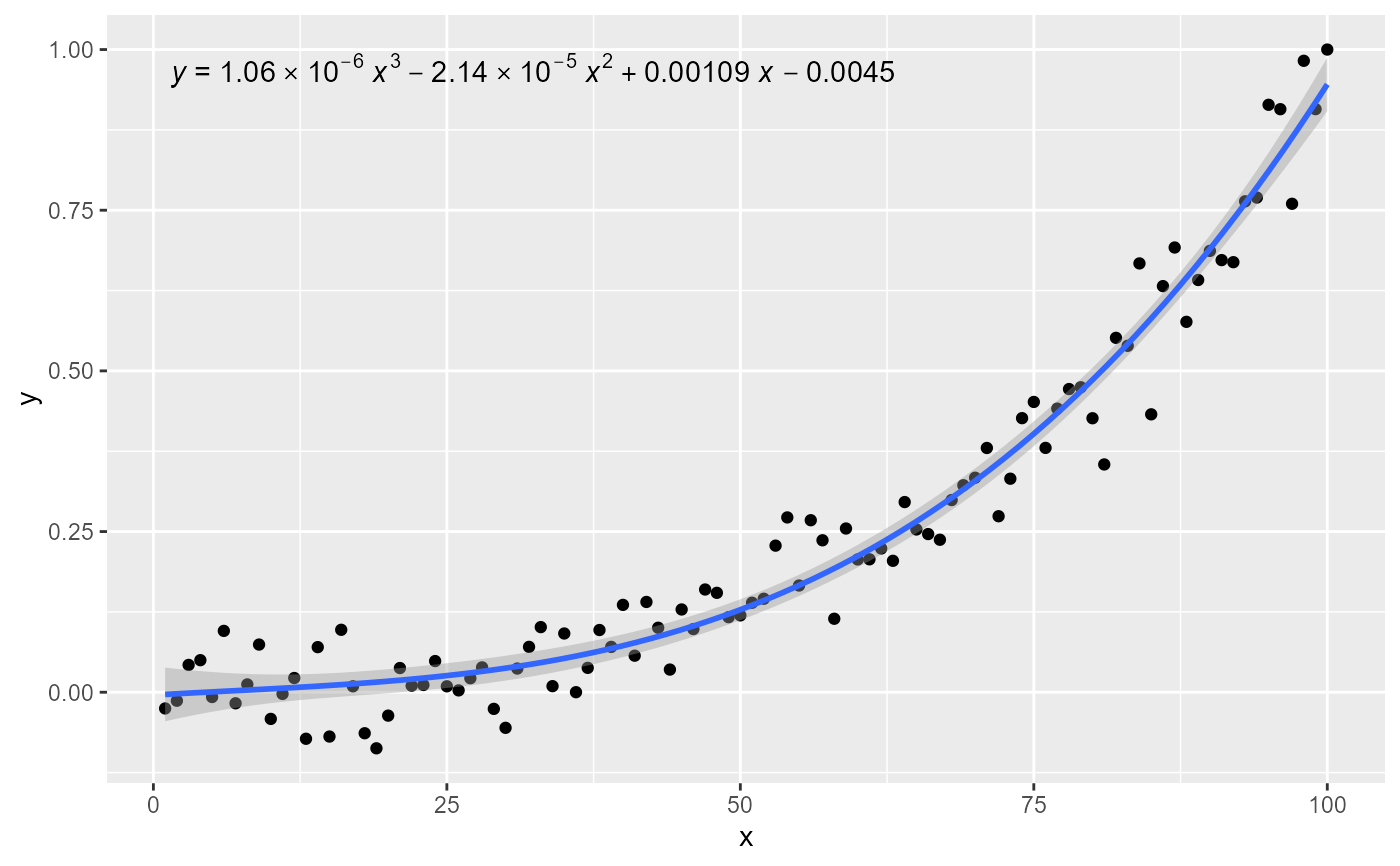# no weights, digits for R square
ggplot(my.data, aes(x, y)) +
geom_point() +
geom_smooth(method = "lm", formula = formula) +
stat_poly_eq(formula = formula, rr.digits = 4, parse = TRUE)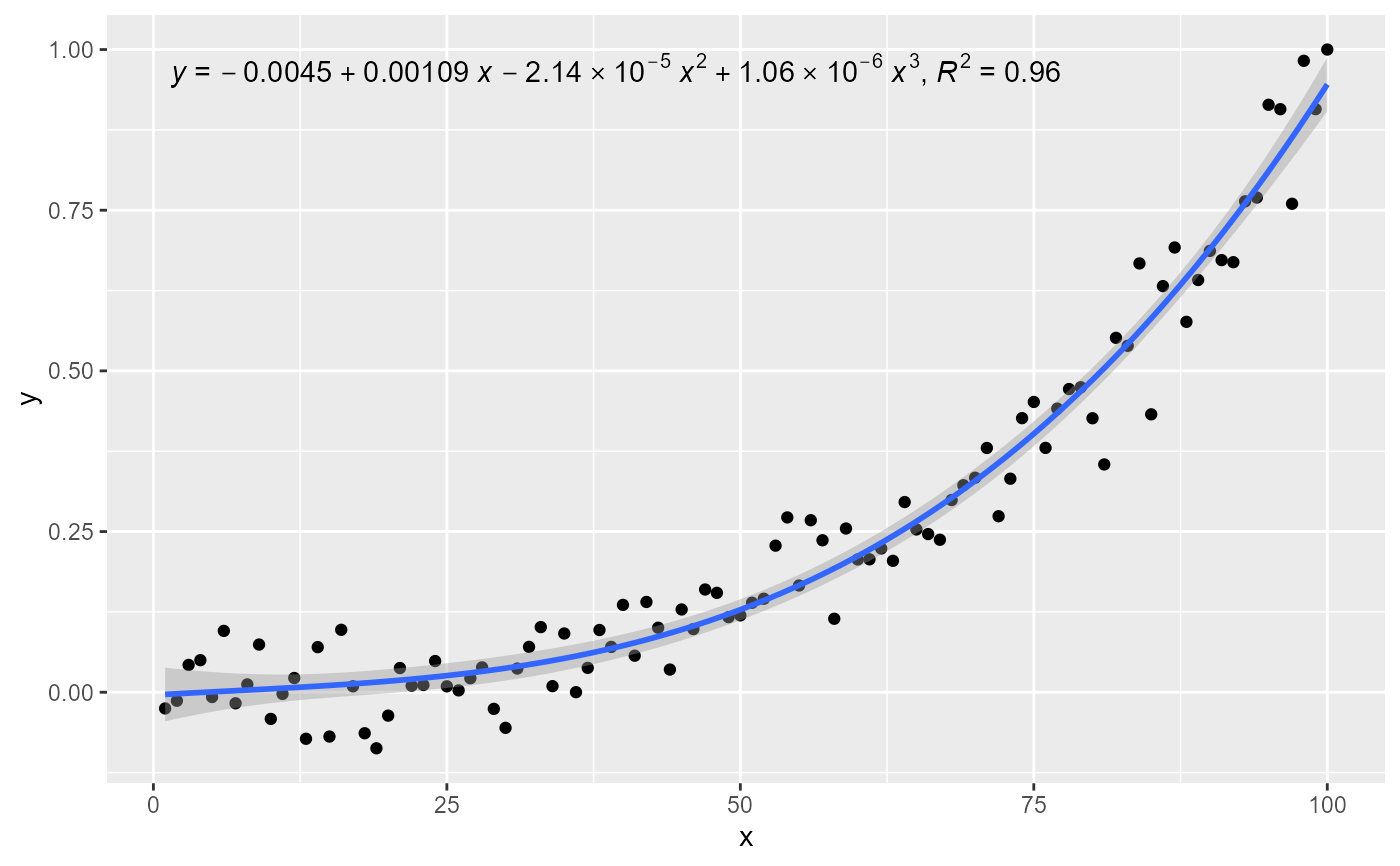# user specified label
ggplot(my.data, aes(x, y)) +
geom_point() +
geom_smooth(method = "lm", formula = formula) +
stat_poly_eq(aes(label =  paste(stat(eq.label), stat(adj.rr.label), sep = "~~~~")),
formula = formula, parse = TRUE)# user specified label and digits
ggplot(my.data, aes(x, y)) +
geom_point() +
geom_smooth(method = "lm", formula = formula) +
stat_poly_eq(aes(label =  paste(stat(eq.label), stat(adj.rr.label), sep = "~~~~")),
formula = formula, rr.digits = 3, coef.digits = 2, parse = TRUE)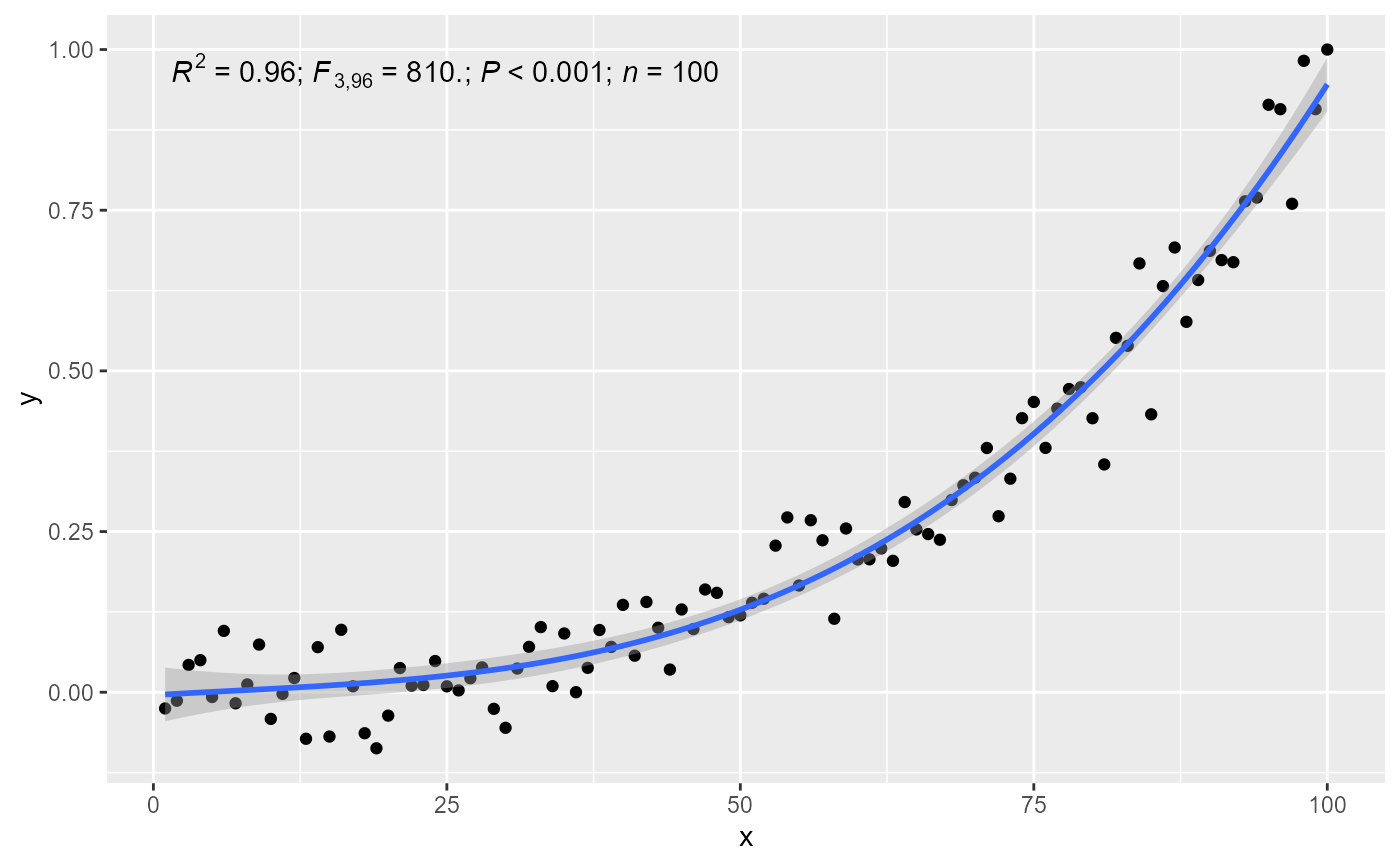# geom = "text"
ggplot(my.data, aes(x, y)) +
geom_point() +
geom_smooth(method = "lm", formula = formula) +
stat_poly_eq(geom = "text", label.x = 100, label.y = 0, hjust = 1,
formula = formula, parse = TRUE)